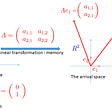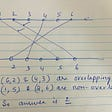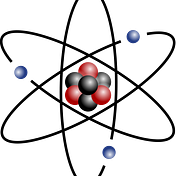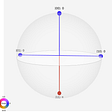# Quantum Algo: Grover Algorithm

In the previous introductory articles, we have discussed about the inversion about mean, amplitude amplification and Grover Iterator.

Setup:

The quantum search algorithm performs a generic search for a solution to a very wide range of problems. Consider any problem where one can efficiently recognize a good solution and wishes to search through a list of potential solutions in order to find a good one.

Define a function as shown below

f(x) = 1 if x is the binary encoding of a ‘good’ string’ (i.e. a solution to the search problem), and f(x) = 0 otherwise(x’ -xprime).

The Search Problem:

Input: A black box Uf for computing an unknown function f (defined above).

Problem: Find an input x such that f(x) = 1.

In the following sections we look at the classical solution and quantum solution.

Classical Algorithm:

As mentioned in the code block, the classical form of search algorithm takes a parameter “user_input”(the user input could be mouse click or voice search or any type of input method). In the current directory the search algorithm performs the search to find the matching file name and returns file if a file name matches else returns file not found.

In the current example there are 8 different types of files exists in the mentioned directory. if the “user_input” matches with any one file name then the search stops and returns the file.

suppose, we consider that searching for an existing file only then

1. in the worst case scenario, the file could match with the last element(N) in the list. In our case it is 8.
2. in the best case scenario, the file could match with the first element in the list.
3. with the best luck trail it could be at N/2 position.

to test the above search algorithm below is the sample test code.

Conclusion: With the classical search algorithm the maximum calls to the Oracle requires N.

Q. Can we perform better than N?

Quantumly, yes!

With the Grover’s search algorithm the maximum calls to the Oracle requires less than or equal to √N (square root of N). Which is a quadratic speed up comparatively.

Quantum Algorithm:

Following are the list of articles we can conclude that grover’s search algorithm requires only √N calls to the oracle.

now let’s have a look at what is the exact value of t for a known N.

Here N represents the total number of elements in a list or in a given set.

The number of qubits(n) can be calculated as length of the given bit string or log N

for now we consider about sine value only

from the same article we can observe that

then by rewriting the the above equation as follows

after substituting the theta value we get as follows

Note: refer conclusion at the bottom for the updated python code for t.

this gives the exact value of t which is the required number of iterations.

In classical algorithm we have considered the text data as values and also input manipulated in the text format.

To work with quantum algorithm we consider the numeric way of representation (text format consumes to many qubits and currently at the time of writing this article we have limited number of qubits).

we represent the elements in the following list as mentioned below

we assign a number to each of the file name as shown below in the numeric and it’s binary form,

numeric form : [0, 1, 2, 3, 4, 5, 6, 7]

binary representation : [000, 001, 010, 011, 100, 101, 110, 111].

As we discussed in the classical algorithm the “user_input” is “groveralgorithm” and it’s equivalent binary representation is 010.

Using IBMQ we solve this problem in the next section.

Implement Grover’s Search Algorithm using Qiskit and Cirq:

In this section we implement Grover’s Search Algorithm circuit using qiskit for n = 3(N = 2³) and using cirq for n= 6(N = 2⁶) where n represents the number of qubits or it is the length of the input bit string.

Implement Grover’s Search Algorithm using Qiskit:

the below function generates initial conditions for the GS algorithm

the below function generates Oracle for the given input.

the below function generates inversion about the mean circuit, here we are using HXH = Z which can be accomplish by using the mcx.

the below function generates operator circuit for the given function.

the below function generates grover search algorithm circuit for the given input with t iterations.

the below code block initializes the circuit with required inputs and draws the final output circuit.

final circuit

the below code block performs measurement using Qasm Simulator

Output histogram

Implement Grover’s Search Algorithm using Cirq:

the below code block imports the required libraries

the below function generates initial conditions for the GS algorithm

the below function generates Oracle for the given input.

the below function generates inversion about the mean circuit, here we are using HXH = Z which can be accomplish by using the multi controlled not gate.

the below function generates operator circuit for the given function.

the below function generates grover search algorithm circuit for the given input with t iterations.

the below code block initializes the circuit with required inputs and draws the final output circuit.

final circuit

the below code block performs measurement using cirq Simulator

Conclusion:

How we are going to construct a circuit with t = 3.5 or 4.6 or any non-zero float value?

Effective and direct answer. No!. Since we cannot construct floating point depth circuit. In the below figure the effective t is 3.7 rotations.

But, there is a chance we can reach to an integer value if we rotate the circuit multiple times over the 360 degrees. Like we rotate 0–360 degrees and 360 to 450 degrees if still we have floating point then repeat until we reach to the integer value.

But this costs in the form of circuit depth and number of gates and time complexity as well.

With a little probability trade-off we can achieve this with ceiling the value to upper bond if the decimal point is 5 or greater than 5 and flooring the value if less than 5. which means we will be choosing the nearest integer away from the chosen part of wave function.

python code used while calculate t in the above main part of the article have used only ceil which is not accurate.

In python, ceil and floor condition can be managed by using the round function as shown below,

Updated: (19/feb/2022) Added conclusion and added modified python code for t in the conclusion.

references: qiskit textbook, cirq tutorials, IITM course by (@Prabha M, Anil Prabhakar), Qubit by Qubit(The Coding School) course, IBM Quantum Challenge 2021, QWorld (QBronze 2021), Wikipedia, stack exchange and open source materials.

--

--

--

## More from Anonymousket

Love podcasts or audiobooks? Learn on the go with our new app.

## Why You Aren’t Popular## Matrices: Eigenvectors Eigenvalues.## Algorithm/DS Problem#3: Minimum non-overlapping bridges## Monty Hall Problem will drive you crazy## Statistics #01 — Mean, Median, and Mode## Knight’s Yarn## An introduction to Quantum Computing## Quantum Key Distribution## Quantum Teleportation — From Fiction to Reality## GHZ and W Quantum states